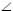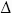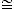# Find the value of x.Hi student,
Please see the diagram for the problem below ,Calculate some known angles:
•ACB = 180-(10+70)-(60+20) = 20°
•AEB = 180-70-(60+20) = 30° Draw a line from point D parallel to AB, labeling the intersection with BC as a new point F and conclude:
•DCFACB
•CFD =CBA = 60+20 = 80°
•DFB = 180-80 = 100°
•CDF =CAB = 70+10 = 80°
•ADF = 180-80 = 100°
•BDF = 180-100-20 = 60°
Draw a line FA labeling the intersection with DB as a new point G and conclude:
•ADFBFD
•AFD =BDF = 60°
•DGF = 180-60-60 = 60° =AGB
•GAB = 180-60-60 = 60°
•DFG (with all angles 60°) is equilateral
•AGB (with all angles 60°) is equilateralCFA with two 20° angles is isosceles, so FC = FA
. Draw a line CG, which bisectsACB and conclude:
•ACGCAE
• FC-CE = FA-AG = FE = FG
• FG = FD, so FE = FD​​​​​​​ . With two equal sides,DFE is isosceles and conclude:
•DEF = 30+x = (180-80)/2 = 50